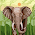# Analysis of Algorithm MCQ's Question with Solution Quiz Interview Final Exam

Data Structure and Algorithm Analysis   Multiple Choice Questions from System Analysis and Design Download the Data structures & Algorithms  you can check this help ful for you  Multiple Choice Questions (MCQs) Questions & Answers Free online for midterm exam for quiz and final exam and for Interview

Q  1.
Here is an array which has just been partitioned by the first step of quick sort:
3, 0, 2, 4, 5, 8, 7, 6, 9
Which of these elements could be the pivot? (There may be more than one possibility!)
5 or 8
Q  2.
Here is an array of ten integers:
5  3  8  9  1  7  0  2  6  4
Draw this array after the TWO recursive calls of merge sort are completed, and before the final merge step has occured.
1 3 5 8 9 and 0 2 4 6 7
Q  3.
Fill in the following table for the times to sort an array of n items. Use only big-O notation, and do not have any extraneous constants in your expressions.
Algorithms Worst Case Average Case
Selection sort n^2 n^2
Insertion sort n^2 n^2
Quick sort n^2 Nlgn
Merge sort Nlgn Nlgn
Binary search of a sorted array lgn lgn

Q  4.
In a selectionsort of n elements, how many times is the swap function called in the complete execution of the algorithm?
a) 1
b) n - 1
c) n log n
d)
Q  5.
Selection sort and quick sort both fall into the same category of sorting algorithms. What is this category?
a) Interchange sorts
c) O(n log n) sorts
d) Divide-and-conquer sorts

Q  6.
Suppose we are sorting an array of ten integers using a some quadratic sorting algorithm. After four iterations of the algorithm's main loop, the array elements are ordered as shown here:
1  2  3  4  5  0  6  7  8  9
Which statement is correct? (Note: Our selection sort picks largest items first.)
a) The algorithm might be either selection sort or insertion sort.
b) The algorithm is neither selection sort nor insertion sort.
c) The algorithm might be selection sort, but could not be insertion sort.
d) The algorithm might be insertion sort, but could not be selection sort.
Q  7.
When is insertion sort a good choice for sorting an array?
a) Each component of the array requires a large amount of memory.
b) The processor speed is fast.
c) Each component of the array requires a small amount of memory.
d) The array has only a few items out of place.
Q  8.
What is the worst-case time for merge sort to sort an array of n elements?
a) O(n)
b) O(n log n)
c) O(log n)
d) O(n²)
Q  9.
What is the worst-case time for quick sort to sort an array of n elements?
a) O(n log n)
b) O(n²)
c) O(log n)
d) O(n)

Q  10. (Marks 1)
Merge sort makes two recursive calls. Which statement is true after these recursive calls finish, but before the merge step?
a) The array elements form a heap.
b) None of the above.
c) Elements in each half of the array are sorted amongst themselves.
d) Elements in the first half of the array are less than or equal to elements in the second half of the array.• Title : Analysis of Algorithm MCQ's Question with Solution Quiz Interview Final Exam
• Posted by :
• Date : 03:05
• Labels :

1.2.